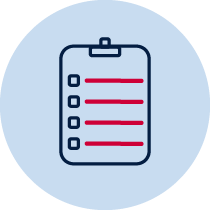# Patterns and algebra – What numbers are missing?Resource required Verbal recording Take photo Teacher observation Individual Whole class

## Numbers and algebra – patterns and algebra 2

• describes mathematical situations and methods using everyday and some mathematical language, actions, materials, diagrams and symbols MA1-1WM
• uses objects, diagrams, and technology to explore mathematical problems MA1-2WM
• supports conclusions by explaining or demonstrating how answers were obtained MA1-3WM
• creates, represents and continues a variety of patterns with numbers and objects MA1-8NA

## Content

Describe patterns with numbers and identify missing elements (ACMNA035)

Solve problems by using number sentences for addition or subtraction (ACMNA036)

Addition and subtraction 2- represent and solve simple addition and subtraction problems using a range of strategies including counting on, partitioning and rearranging parts (ACMNA015)

## National Numeracy Learning Progression mapping to the NSW mathematics syllabus

When working towards the outcome MA1-8NA the sub-elements (and levels) of Additive strategies (AdS6) and Number patterns and algebraic thinking (NPA5) describe observable behaviours that can aid teachers in making evidence-based decisions about student development and future learning.

## Materials

• Large chart paper and pen for teacher recording
• Paper, pencils, whiteboards, pens or recording device
•A range of numberless word problems## Teacher instructions

The purpose of this task is to gauge students’ understanding of concepts such as

• solve problems by using number sentences
• identify missing elements
• describe how a missing number in a number sentence was calculated
• represent a word problem as a number sentence
• pose a word problem to represent a number sentence

This activity can be done with the whole class or a small group.

Display a numberless word problem such as ‘There were some horses in a field. Then some more horses joined them’, on a large piece of paper. Students can think, pair share what they know for example, ’I can see one group of horses and then some more horses came along and made the group bigger.’ Depending on the group, students could draw what they can visualise on a whiteboard. Students need to come up with any questions to determine the information needed to make this into a word problem that can be solved using a number sentence.

Examples of questions for this problem.

How many horses were in the field first? Student example ‘There were 7 horses in the field.’

How many horses are there all together? Student example ‘There were 15 horses all together.’

How many horses were in the second group?

Record student examples and then transform the information into a number sentence.

7 + __ = 15

## Student instructions

Teacher: We are going to solve a numberless word problem. ‘There were some horses in a field. Then some more horses joined them.’

Teacher:What can you visualise or see? Share what you are visualising with a buddy or draw this on your whiteboard. (Record on your chart some examples from students).

Teacher: What information is missing from the word problem? (Students think, pair share). Ask one student to share what we need to know and write this on the chart (example there were 7 horses in the field).

Teacher:Discuss with students that we need to know things to solve problems (use enabling questions What changed? What did we learn from this new information? What question could we ask about this situation?)

Teacher: (Students to think, pair share). What other information could we include? (example there were 15 horses all together. Use enabling questions What changed? What did we learn from this new information? What operation could we use and why? What do we need to find out? Students may discuss using addition or subtraction 15-7 = ?)

7 + 8 = ? result unknown

7 + ? = 15 change unknown

? + 8 = 15 start unknown

Provide students with a variety of numberless word problems to read, think about and record questions and number sentences related to the information in their question.

Students can then create a word problem, with numbers, to share with their peers.

## Possible areas for further exploration

Students:

• could not determine any questions to ask for their numberless word problem
• could devise one result question for their numberless word problem
• could create two questions to match their numberless word problem
• could create three questions and transform these into processes to create a word problem for a peer. Word problems contained a combination of start, change or result unknown.
• could transform their peer’s word problem into a number sentence

## Where to next?

• Explicit teaching on developing number sentences involving one operation and creating word problems to match these. Focus on identified student needs e.g. if students can only solve result unknown then teach start and change unknown for a range of problems.

Syllabus outcomes and content descriptors from Mathematics K-10 Syllabus © NSW Education Standards Authority (NESA) for and on behalf of the Crown in right of the State of New South Wales, 2012